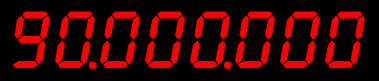Frequency counter

(Please note that this program will not run without a valid GPS signal)

The RFzero system has a built-in high precision frequency counter that can measure up to 90 MHz. The primary purpose of the counter is to measure the Si5351A’s reference frequency (27 MHz) which makes it possible to generate an RF output frequency with a precision much better than 1 Hz. This is simply done  by taking into account the exact frequency of the reference frequency when doing the math for the synthesizer inside the Si5351A.The frequency counter is implemented partly in hardware and partly in software. This means that it is both very accurate and highly configurable. Instead of the reference frequency you can use the frequency counter to count the frequency of a signal present on an input pin. You may even measure the MCU clock if you want.

Characteristics of the frequency counter are

• It cannot be used for stand alone purposes at the same time it is used for the RFzero’s own use, i.e. measuring the reference frequency
• The maximum input signal voltage is 3,6 V
• The minimum input signal voltage is 0 V
• The minimum “high” logic level voltage is 2,0 V
• The maximum “low” logic level voltage is 1,0 V
• A DC coupled input signal must be offset to meet both the “high” and “low” logic levels, i.e. 1,6 V for a symmetric input signal
• The maximum measurable frequencies are
• 90 MHz: square wave and DC coupled
• 65 MHz: sinusoidal and AC coupled
• The frequency resolution is 1 s due to using the 1 PPS from the GPS, but, averaging increases the precision
• Measuring the frequency of a signal on a pin is limited to pins D3, D8, D9 and D13

By default the frequency counter program is setup to measure the frequency of a signal on D8.

While measuring the signal the result is output to the USB port or LCD. In addition to the measured frequency the counter also calculates the average frequency over up to 128 x 1 s periods. Also the standard deviation is calculated.

Display

The display is a LCD, HD44780 20×4 parallel only, and is highly recommended, because it gives a better overview than the serial terminal does.

The LCD shows the latest frequency measurement, the average frequency that is averaged over up to 128 periods and the standard deviation. If the GPS PPS is present the LCD shows “GPS” otherwise this is off.If you want to change what is shown on the display please edit the // Prepare LCD section in the setup() function and in the // Print … sections in the loop() function in the FrequencyCounter.ino file.

Theory behind the frequency counter

The frequency counter is made by counting the number of positive/rising edges of the digital signal for a certain period. If this period is one second then the number of edges counted is the same as the frequency of the signal. The PPS from the GPS is a signal that is perfect for this type of application. The PPS goes from low to high exactly ones per second and when this happens it can start or stop the counting – in the professional world this is called the gate signal of the frequency counter.

Principal block schematic of the RFzero built-in frequency counter.Thus the procedure for the frequency counter is

1. Initialize GPS
2. Setup the MCU and two pins to receive the signal and the PPS
3. When a PPS arrives start the counter
4. Let the signal increment the counter
5. When a PPS arrives again read the counter then reset the counter and go to 4

According to the datasheet the PPS accuracy of the u-blox NEO-7M is better than 30 ns RMS. Real measurements show that the typical result is better than 1 Hz of a 48 MHz signal. But for all digital measurements it applies that the last digit is always +/-1, e.g. 47 999 999; 48 000 000 or 48 000 001.

How precise is the frequency counter?

The counter software performs some averaging of the measured value, so in general, the longer you let it run, the more accurate it is going to be. There is also some statistics that you can examine to see how precise the measurements are. See the box below how use the statistics.